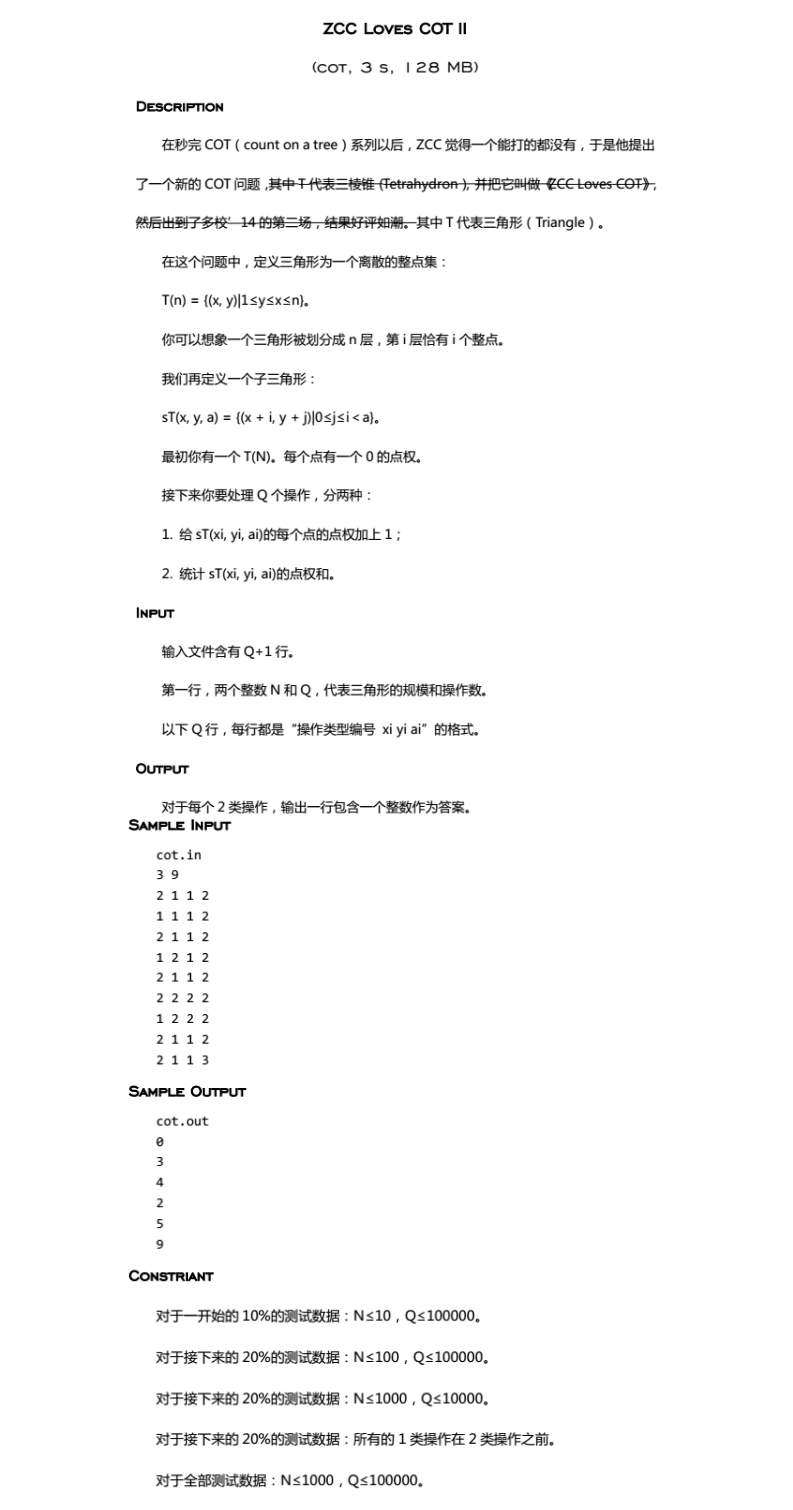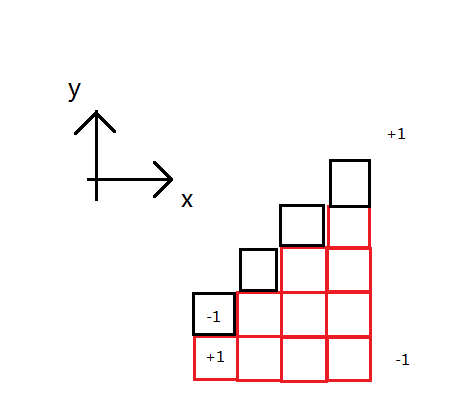$p_{x,y}$表示$(x,y)$点权

$R_{x,y}=\sum\limits_{i=1}^{x}\sum\limits_{j=1}^{\min(y,i)}p_{i,j}$
$T_{x,y}=\sum\limits_{i=x-y+1}^{x}\sum\limits_{j=1}^{\min(y,i)}p_{i,j}$#include<algorithm>
#include<iostream>
#include<cstring>
#include<cstdio>
#include<cmath>
#define LL long long
#define M 1010
#define BC 2500
#define mid ((l+r)>>1)
using namespace std;
LL nm=0,fh=1; char cw=getchar();
for(;!isdigit(cw);cw=getchar()) if(cw=='-') fh=-fh;
for(;isdigit(cw);cw=getchar()) nm=nm*10+(cw-'0');
return nm*fh;
}
int tg1[M][M],tg2[M][M],p1[M][M],p2[M][M],qx[M<<2],qy[M<<2],qd[M<<2],cnt;
int F[M][M],val[M][M];
LL R[M][M],T[M][M];
void solve(){
for(int i=1;i<=n;i++){
for(int j=1;j<=i;j++){
p1[i][j]+=p1[i-1][j],p2[i][j]+=p2[i-1][j-1];
F[i][j]=F[i][j-1]+p1[i][j]+p2[i][j],val[i][j]+=F[i][j];
R[i][j]=R[i-1][j]+R[i][j-1]-R[i-1][j-1],R[i][j]+=val[i][j];
T[i][j]=T[i-1][j-1]+R[i][j-1]-R[i-1][j-1],T[i][j]+=val[i][j];
}
}
memset(p1,0,sizeof(p1));
memset(p2,0,sizeof(p2));
}
LL getans(int x,int y,int k){
LL sum=0;
for(int i=1;i<=cnt;i++){
int flr=max(y,qy[i]),rs=min(x+k-1,qx[i]+qd[i]-1);
int ctx=max(x-y,qx[i]-qy[i]); LL len=rs-(ctx+flr)+1;
if(len>0) sum+=((len*(len+1))>>1);
} return sum;
}
int main(){
if(tpe==1){
++cnt,qx[cnt]=x,qy[cnt]=y,qd[cnt]=dt;
p1[x][y]++,p1[x+dt][y]--,p2[x][y+1]--,p2[x+dt][y+dt+1]++;
if(cnt==BC) solve(),cnt=0; continue;
}
LL ans=T[x+dt-1][y+dt-1]-T[x-1][y-1];
ans-=R[x+dt-1][y-1]-R[x-1][y-1];
ans+=getans(x,y,dt),printf("%lld\n",ans);
}
return 0;
}


## Cot的更多相关文章

1. spoj cot: Count on a tree 主席树

10628. Count on a tree Problem code: COT You are given a tree with N nodes.The tree nodes are number ...

2. spoj COT - Count on a tree (树上第K小 LCA+主席树）

链接: https://www.spoj.com/problems/COT/en/ 思路: 首先看到求两点之前的第k小很容易想到用主席树去写,但是主席树处理的是线性结构,而这道题要求的是树形结构,我们 ...

3. cos,sina,tan,cot

这些都是三角形的公式. 三角形总之就是一个直角两个锐角. cos就是余弦定理,就是锐角的邻边除以三角形的斜边. sin就是正弦定理,就是锐角的对边除以三角形的斜边. tan就是正切定理,就是锐角的对边 ...

4. Count on a tree（SPOJ COT + 树上第k大 + 主席树 + LCA）

题目链接:https://www.spoj.com/problems/COT/en/ 题目: 题意: 给你一棵有n个节点的树,求节点u到节点v这条链上的第k大. 思路: 我们首先用dfs进行建题目给的 ...

5. SPOJ - COT Count on a tree

地址:http://www.spoj.com/problems/COT/en/ 题目: COT - Count on a tree #tree You are given a tree with N  ...

6. 正割、余割、正弦、余弦、正切、余切之间的关系的公式 sec、csc与sin、cos、tan、cot之间的各种公式

1.倒数关系 tanα ·cotα＝1 sinα ·cscα＝1 cosα ·secα＝1 2.商数关系 tanα=sinα/cosα cotα=cosα/sinα 3.平方关系 sinα²+cosα ...

7. SPOJ 10628 COT - Count on a tree（在树上建立主席树）（LCA）

COT - Count on a tree #tree You are given a tree with N nodes.The tree nodes are numbered from 1 to ...

8. 【学术篇】SPOJ COT 树上主席树

这是学完主席树去写的第二道题_(:з」∠)_ 之前用树上莫队水过了COT2... 其实COT也可以用树上莫队水过去不过好像复杂度要带个log还是怎么样可能会被卡常数.. 那就orz主席吧.... 写了 ...

9. c/c++ 实现三角函数（不使用库函数） sin/cos/tan/cot

c/c++ 实现三角函数(不使用库函数) sin/cos/tan/cot #include <iostream> #include <cstdlib> #include < ...

10. 【填坑向】spoj COT/bzoj2588 Count on a tree

这题是学主席树的时候就想写的,,, 但是当时没写(懒) 现在来填坑 = =日常调半天lca(考虑以后背板) 主席树还是蛮好写的,但是代码出现重复,不太好,导致调试的时候心里没底(虽然事实证明主席树部分 ...

## 随机推荐

1. React antd嵌入百度编辑器（css加载不到等问题，'offsetWidth' of null）

之前有看过一些类似的文章,以为嵌入不会遇到太多坑 结果...    其他不说,先来描述下跳坑的过程 先定义Ueditor.js类,这个和网上版本类似 import React, { Component ...

2. Android插件化(使用Small框架)

github: https://github.com/cayden/MySmall Android插件化(使用Small框架) 框架源代码 1. Create Project File->New ...

3. B. Worms Codeforces Round #271 (div2)

B. Worms time limit per test 1 second memory limit per test 256 megabytes input standard input outpu ...

4. 反射机制，jvm，class类型

[说明]这是上午完成的内容或者说是接触到的知识点,包括servlet简单的数据库连接,表格的显示需要用到的插件jstl,还有最最多的java反射原理的讲解 1)数据库的设计 2)编程中用到的知识点 3 ...

5. 【BZOJ3563/3569】DZY Loves Chinese II 线性基神题

[BZOJ3563/3569]DZY Loves Chinese II Description 神校XJ之学霸兮,Dzy皇考曰JC. 摄提贞于孟陬兮,惟庚寅Dzy以降. 纷Dzy既有此内美兮,又重之以 ...

6. 自然常数e的神奇之美

7. php自定义函数: 遍历文件夹及其子文件夹

function traverse_folder($path = '.') {$current_dir = opendir($path); while(($file = readdir(\$curre ...

8. CXF生成client注意事项

1. 在使用wsdl2java命令生成client文件时在Service的Java文件中面出现super构造错误,这是因为jax-ws2.2规约与java6冲突  故须要减少jax-ws规约版本号. ...

9. Linux中各种压缩文件

.gz格式 压缩: gzip 文件名 解压: gzip -d 欲解压文件名 gunzip 欲解压文件名 说明: 1.只能压缩文件,不能压缩目录 2.压缩和解压的时候不保留原文件 .bz2格式 压缩: ...

10. mysql 分页测试，

大环境:MySQL5.6 自己造了 27万数据, 一次性 查出来,会超时: 而分页跑,会查出来8s: 但是在少于27万时,直接查比 分页查快5倍: